﻿ MotionDesigner Reference & User Interface > Motion Laws / Cam-Laws for Segments > Y-Inverse-Sinusoid [Special]

# Motion-Law: Y–Inverse-Sinusoid

### Y–Inverse-Sinusoid

Note: The Y-Inverse-Sinusoid motion-law is usually applied to a rotating part, e.g. a Crank. There can be only one Y-Inverse-Sinusoid segment in each motion.

The Constant-Velocity Inverse Crank is a similar motion-law. There can be more than Constant-Velocity Inverse Crank one segment in each motion.

Motion-Description

 We can explain this motion-law if we project the motion of a Point that is at the end of a rotating Crank onto a Line. When a Crank rotates with Constant angular velocity, the motion of a Point that we project onto any Line is Simple-Harmonic-Motion. When the Crank rotates with the Y-Inverse-Sinusoid motion-law, the motion of a Point that we project onto a particular Line is Constant Linear Velocity.

#### Motion-Values

You CAN control the

 Start-Position - defined by the three Segment-Parameters - see below. End-Position - defined by the three Segment-Parameters - see below.

You CANNOT control the:

 Velocity at the Start or End Acceleration at the Start or End Jerk at the Start or End See Note below, and example.

Segment Parameters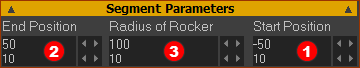Example Segment Parameters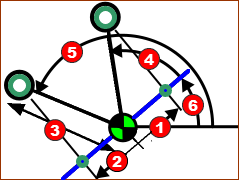Schematic of Crank made to give a constant linear velocity ... Angle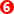is to Blue line relative to any other line, as defined by the Base-Value of the Motion-Dimension FB. Radius of Rocker (Example is 100mm) The Radius of the Rocker (rotating-Part) specifies the minimum and maximum distance from the rotating-axis of the Green point, at the end of the Rocker, projected onto the Blue line. Start-Position(Example SP=–50mm) Directional Distance from the rotating-axis of the Green point projected onto the Blue line when the Green point starts to move with Constant-Velocity along the Blue line. End-Position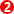(Example EP = 50mm) Directional Distance from the rotating-axis of the Green point projected onto the Blue line when the Green point ends moving with Constant-Velocity along the Blue line. We calculate for you the angles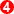and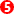for the Rocker relative to the Blue Line, from the Start-Position and End-Position. Note: The Crank rotates Counter-Clockwise when Start-Position is a Negative and End-Position is a Positive. The Crank rotates Clockwise when End-Position is a Negative and Start-Position is a Positive.

Segment-Range

 Start-Range End- Range 0 ≤ Start-Range < End-Range ≤ 1Video: Y-Inverse-Sinusoid Example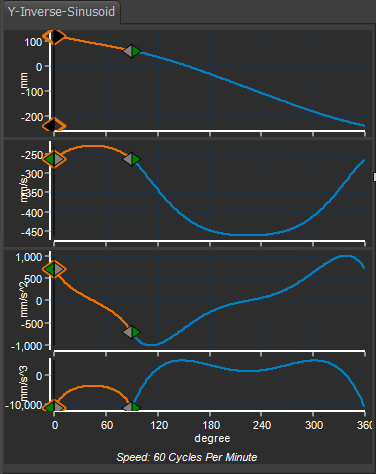Y-Inverse Sinusoid

Example: with two segments.

Crank rotates in a Counter-Clockwise direction

Constant-Velocity Starts at 50mm to Left of Crank-Center (angle 120º)

Constant-Velocity Ends at 50 to Right of Crank-Center (angle 60º)

Segment 1:

X-axis Start = 0 ; End = 90º

The Y–Inverse Sinusoid Segment-Parameters are:

Radius of Rocker = 100mm, Start Position = -50, End Position = 50mm.

With these Segment-Parameters:

The Y–axis is 120º†, when the Master Machine Angle (MMA) is 0º .

The Y–axis is 60º, when the Master Machine Angle is 90º .

Segment 2:

X-axis Start = 90º, End = 360º (0º)

A Flexible-Polynomial

The Y–axis is 60º, when the Master Machine Angle is 90º (= end of Segment 1)

The Y–axis –240º when the Master Machine Angle is 360º .

Thus the Crank makes a full rotation from +120 to -240 in a counter-clockwise direction.